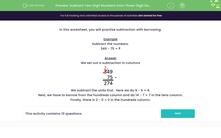# Use Borrowing to Subtract Two-Digit Numbers from Three-Digit Numbers

In this worksheet, students will subtract two-digit numbers from three-digit numbers using a written column method.Key stage:  KS 2

Curriculum topic:   Number: Addition and Subtraction

Curriculum subtopic:   Add/Subtract up to Four-Digit Numbers

Difficulty level:#### Worksheet Overview

In this activity, we will practise subtraction with borrowing.

Example

Subtract the numbers.

349 - 75 = ?

We set out a subtraction in columns.We subtract the ones first.  Here we do 9 - 5 = 4.

Next, we have to borrow from the hundreds column and do 14 - 7 = 7 in the tens column.

Finally, there is 2 - 0 = 2 in the hundreds column.

Don't worry if this seems a bit tricky, we can do plenty of practice in the questions that follow.If you want to look back at this example again, just click on the red help button that appears on the side of the screen during the questions.

### What is EdPlace?

We're your National Curriculum aligned online education content provider helping each child succeed in English, maths and science from year 1 to GCSE. With an EdPlace account you’ll be able to track and measure progress, helping each child achieve their best. We build confidence and attainment by personalising each child’s learning at a level that suits them.

Get started